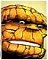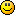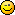You are not logged in.

## #1 2009-08-21 01:14:53

jespa
Member### Firewii 1.0 source code

So, as I promised in this post I am going to release the source code I wrote test the GRRLIB_Plot() and GRRLIB_Line() for GRRLIB 4.1

It is a demo effect used by Denthor in its Asphyxia tutorial #19, written in 1996. He had to write the code in assembler to be able to simulate a fire of 80x110 pixels on a 80286.  Today, we can do a fullscreen demo (640x480) on a Wii if we use some optimization techniques. The effect has something hypnotic, and has some parameters that allow to change the fire which makes it even more interesting (use with caution, pleaseGRRLIB staff is free to distribute the source code with GRRLIB if consider that has interest for demo, or use it as part of a intro. The binary has been released in wiibrew this morning

The current version has some interesting optimization (use of << and >> to avoid divisions, and use of & to avoid module operations, etc...) but can get ever more optimizations. For example, use pointer arithmetic to avoid those matrix[x][y]+matrix[x][y-1]+matrix[x-1][y-1]+matrix[x+1][y-1] stuff, or those rand() calls inside the simulation step. There is a lot of work we can do about it, but the cost is that the source will be less legible. Good for an intro, but bad as a learning example.

So if you are interested in distributing it in the grrlib/examples directory, I would prefer to write and publish several sources ( 0. Not optimiced, 1. How to avoid integer multiplication and division, 2. Pointer arithmetic 3. A burning pirate that moves on the screen, 4. How to plot directly on framebuffer, etc...)

I hope you enjoy the code and give me any comment you have.

Regards.

#### Code:

```/****************************************************************************
Fire animation demo for Wii.
Written by Jespa in 2009
Based on Asphyxia VGA tutorial #19, written by Denthor a long long time ago.
Version 1.0 (a.k.a. Just works!). Can be heavily optimized.
****************************************************************************/

#include <stdlib.h>
#include <stdio.h>
#include <string.h>
#include <gccore.h>
#include <grrlib.h>

Mtx GXmodelView2D;

//This vector stores the RGBA color used to draw the fire.
u32 fireColors;

//fireMatrix keep the current state of simulation. Each dot in the matrix stores the temperature of air
//using a range of 200 values
#define fireMatrix_W 320
#define fireMatrix_H 235
u32 fireMatrix[fireMatrix_H+1][fireMatrix_W];

//Temperature decreasing factor on each simulation step
int fireCombustion = 3;

//Flag to indicate a temporally extra hot fire.
int extraFire=0;

//Draw fireMatrix using the colors given in fireColors
//The matrix will be scaled so each point plot 4 pixels in the screen.
void fireMatrix_Draw(int sx, int sy)
{
int x, y;
sy += 2*fireMatrix_H;
for (y=1; y< fireMatrix_H; y++)
{
for (x=0; x< fireMatrix_W; x++)
{
u32 color = fireColors[fireMatrix[y][x]];

//Plot a pixel and the pixel above of it
GRRLIB_Plot(sx+x,sy-2*y-1,color);
GRRLIB_Plot(sx+x,sy-2*y,color);

//Instead of plot aside the previous pixels, we plot in the other half
//of screen, so we dont see huge 4x4 pixel blocks
GRRLIB_Plot(sx+x+fireMatrix_W,sy-2*y-1,color);
GRRLIB_Plot(sx+x+fireMatrix_W,sy-2*y,color);
}//for
}//for

}//void fireMatrix_Draw(int sx, int sy)

//Makes one step of fire animation.
void fireMatrix_Anim()
{
int x, y;
register int acc;

//Botton line is build using random noise. If extraFire is set, adds extra hot to each dot.
for (x=0; x< fireMatrix_W; x++)
{
if (extraFire > 0)
fireMatrix[x] += rand() & 255;
else
fireMatrix[x] = rand() & 255;
}

//Rest of matrix is build from interpolation. Each dot temperature is averaged
//with its three inferior neighbours and a little noise is added.
//If extraFire is set, adds extra hot to each dot.
for (y=1; y<fireMatrix_H;y++)
{
for (x=0; x< fireMatrix_W; x++)
{
//Adds the value of this dot, plus 3 neighbours, plus extra noise.
acc =   fireMatrix[y][x] +
fireMatrix[y-1][x+1] +
fireMatrix[y-1][x] +
fireMatrix[y-1][x-1]+
(rand() & ((extraFire > 0)?31:15));

//Divide the result by 4, to compute a valid average
acc >>= 2;

//Decrease the value due to combustion
if (acc > fireCombustion)
acc -= fireCombustion;
else if (acc > 0)
acc--;

//Check for overflows due to noise.
if (acc > 199)
acc = 199;

fireMatrix[y][x] = acc;
}//for

}//for

//Decrease the value of extraFire.
if (extraFire > 0)
extraFire--;

}//void fireMatrix_Anim()

//Create the RGBA colors used to draw the fireMatrix.
void fireColors_Init()
{

int conta=0;
int i=0;
register u32 color;

//First section. From (0,0,0) to (63,33,0) in 126 steps
for (i=0; i<126;i++)
{
if ((i & 1) && (conta < 63) )
conta++;

color = 0x000000FF;                          //A
color |= conta << 24;                        //R
color |= ((conta>30)?(conta-30):0)  << 16;   //G
color |= ((conta>8)?((63-conta)>>2):0) << 8; //B
fireColors[i] = color;
} //for

//Second section. From (63,33,0) to (126,64,63)
conta=0;
for (i=126; i<255;i++)
{
if ((i & 1) && (conta < 63) )
conta++;

color = 0x000000FF;             //A
color |= (63+conta) << 24;      //R
color |= (33+conta/2) << 16;    //G
color |= conta << 8;            //B
fireColors[i] = color;
}//for

} //fireColors_Init

//Draw the fireColors values. This is only for testing and debug.
void fireColors_Draw(int sx, int sy, int width)
{
int c;
for (c=0; c<255; c++)
GRRLIB_Line(sx,sy+c,sx+width, sy+c, fireColors[c]);
}//void fireColors_Draw(int sx, int sy)

int x= 50;
int y = 100;

//The one and only... main function!!!
int main() {

u32 timeout=0;

//Init libraries
GRRLIB_Init();

//Init the fireMatrix and fireColors structures
fireColors_Init();
memset(fireMatrix, 0, sizeof(fireMatrix) );

//Clear the screen
GRRLIB_FillScreen(0x000000FF);

//Animation loop. It comes with two different flavours
//while(timeout++ < 4000) { //Exit after a given number of iteration.
while(1) {                  //Run until user press home button in the pad.

//Animate and draw the fireMatrix
fireMatrix_Anim();
fireMatrix_Draw((640-2*fireMatrix_W)>>1, (480-2*fireMatrix_H)>>1);

//Draw the fireColors values. This is only for testing and debug.
//fireColors_Draw(1, 100, 50);

//Ask GRRLIB to show show changes on screen
GRRLIB_Render();

//Miramos si el mando está siendo agitado

//Check for button activity in wpad
{
//Any button activity will restart the count to timeout
timeout=0;

//HOME button will exit from the animation loop
break;

//UP button will decrease the fireCombustion factor
if (fireCombustion>1)
fireCombustion--;

//DOWN button will increase the fireCombustion factor.
fireCombustion++;

//LEFT button will reset the fireCombustion factor
fireCombustion=3;

//A button will add extraFire for a time period
extraFire+=7;

}//Animation loop end.

// Be a good boy, clear the memory allocated by GRRLIB
GRRLIB_Exit();
exit(0);

}// int main()```

Last edited by jespa (2009-08-24 15:51:40)

Offline

## #2 2009-08-21 12:10:03

BlueChip
Moderator

### Re: Firewii 1.0 source code

Nice demo dudePersonally, I think that if the program is on WiiBrew, then the source code should also be there.  Of course, that does not preclude it being in this forum or in the GRRLIB SVN (the GRRLIB demo is released separately).

Maybe, instead of lots of seperate demos, a single demo with "press A for the other algorithm"?  Just a thought.

I have a random number generator based on linear incongruency which reduces to couple of instructions.  You can grab it on this page, it has full documentation and biblio
http://homepage.ntlworld.com/cyborgsyst … ockBox.htm
...it's useless for crypto, but produces a starfield indistinguishable from that generated by a Marseinne Twister ...You will notice that the random'ness happens in the MSb's, so taking 1/n will give you a good random spread over 32bits

BC

I can be found on efnet, freenode, msn, gtalk, aim, ychat & icq ...PM me for details

Offline

## #3 2009-08-21 13:18:01

jespa
Member### Re: Firewii 1.0 source code

Thanks Bluechip,

I know about random number generator based on linear incongruency, which are perfect for this. But I preferred  to do the source more "didactic" using just essential code. I'm not sure if libogc or grrlib has a fast rand() alternative, but if not we could think about a u32_rand(), u16_rand() and f_rand(). We could read wiimote accelerometers noise to build a random seed on grrlib_Init()And I agree  that it is no good to create lots of independent demos. I was speaking about a "basic optimization course" in the same way as this post about modulo arithmetic. Again, it is better to publish changes in the code in the forum than provide lots of versions in the GRRLIB/examples directory

And I suppose that we can rewrite the code to "burn" a texture, so get something as this.

void GRRLIB_BMFX_Burn(const GRRLIB_texImg * texsrc, GRRLIB_texImg *texdest, const u32 factor)

Offline